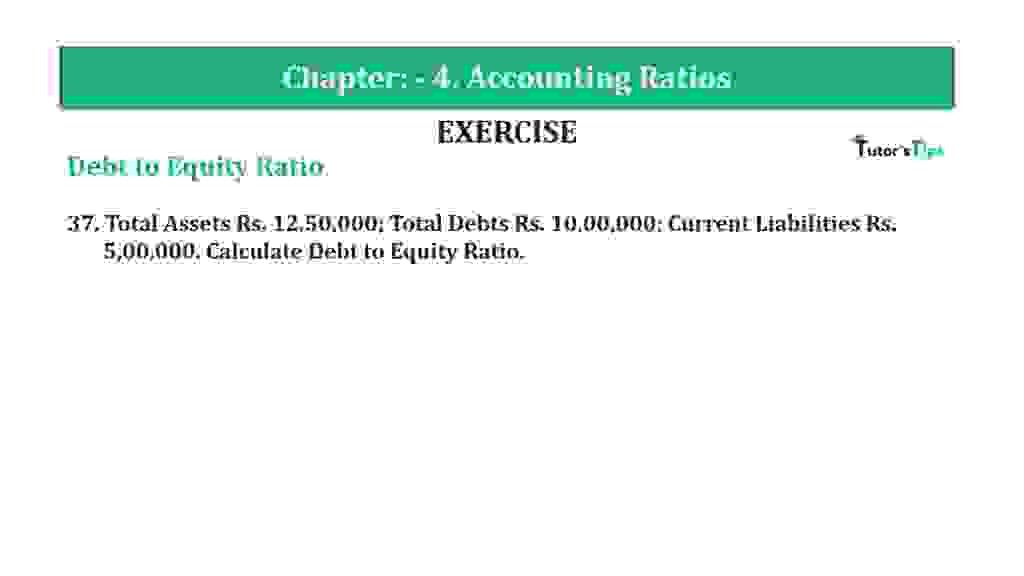# Question 37 Chapter 4 of +2-B – T.S. Grewal 12 ClassQuestion 37 Chapter 4 of +2-B

37. Total Assets Rs. 12,50,000; Total Debts Rs. 10,00,000; Current Liabilities Rs. 5,00,000. Calculate Debt to Equity Ratio.

### The solution of Question 37 Chapter 4 of +2-B: –

 Total Assets = Rs. 12,50,000 Total Debts = Rs. 10,00,000 Equity = Total Assets –  Total Liabilities = Rs.12,50,000 –  Rs.10,00,000 Equity = Rs.2,50,000 Long-term Debts = Total Debts –  Current Liabilities = Rs.10,00,000 –  Rs.5,00,000 Long-term Debts = Rs. 5,00,000

 Debt to Equity Ratio = Long-term Debts = Rs.5,00,000 Equity Rs.2,50,000 = 2: 1

Balance Sheet: Meaning, Format & Examples

Thanks, Please Like and share with your friends

Comment if you have any question.

Also, Check out the solved question of previous Chapters: –

### T.S. Grewal’s Double Entry Book Keeping (Vol. II: Accounting for Companies)T.S. Grewal’s Analysis of Financial Statements